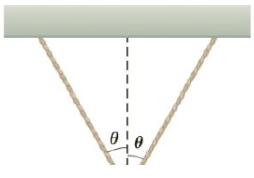Chapter 4, Problem 32P

Chapter
Section
Textbook Problem

Two identical strings making an angle of θ = 30.0° with respect to the vertical support a block of mass m = 15.0 kg (Fig. P4.32). What is the tension in each of the strings?To determine
The tension on each of the strings.

Explanation

Given info: The mass of the block is 15.0 kg. Strings make an angle of 30ο with respect to the vertical support.

The horizontal sine components of tension cancel each other because they are equal in magnitude and opposite in direction. The vertical cosine components get added to balance the weight of the block

From the above diagram,

2Tcosθ=mg

• m is the mass of the block.
• g is the acceleration due to gravity.
• T is the tension on the string.

On re-arranging the terms,

T=mg2cosθ

Substitute 15.0 kg for m, 30ο for θ and 9

Still sussing out bartleby?

Check out a sample textbook solution.

See a sample solution

The Solution to Your Study Problems

Bartleby provides explanations to thousands of textbook problems written by our experts, many with advanced degrees!

Get Started